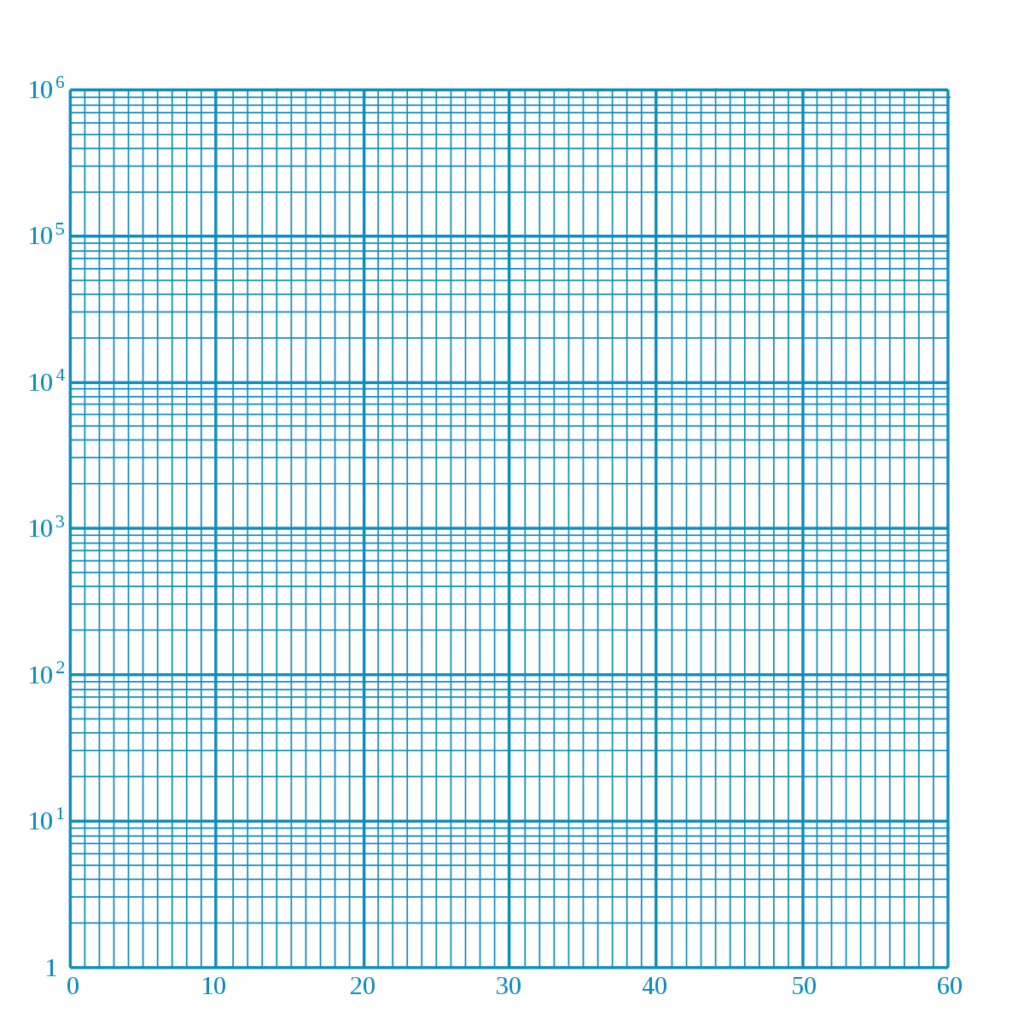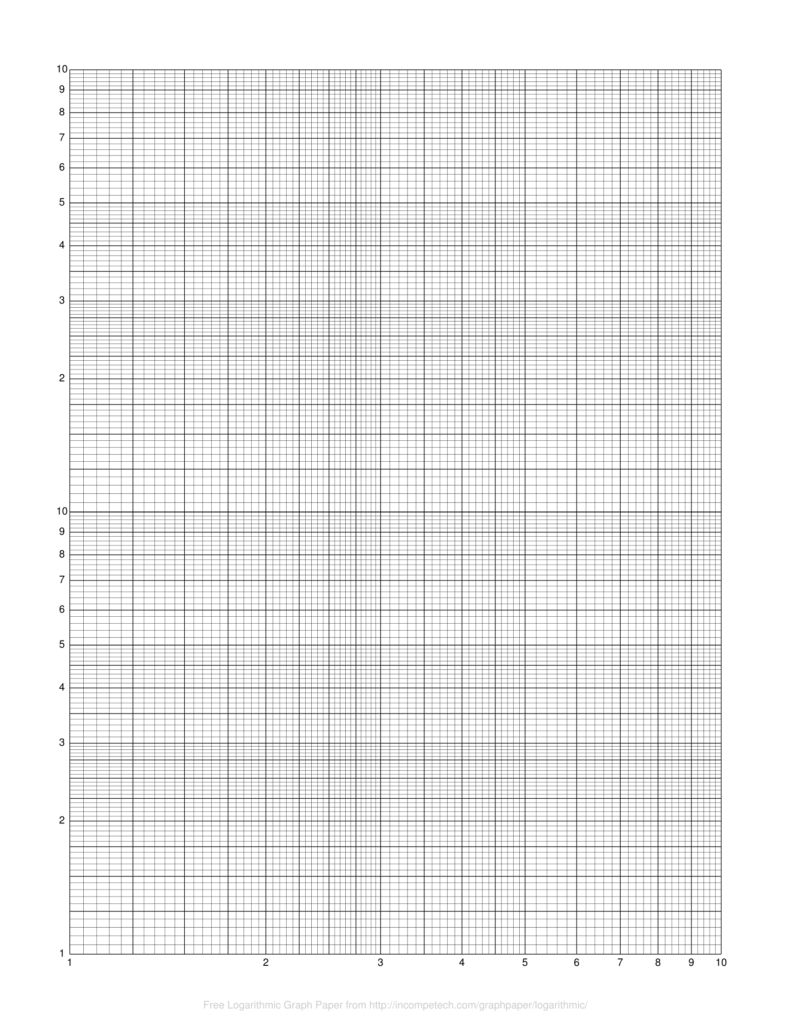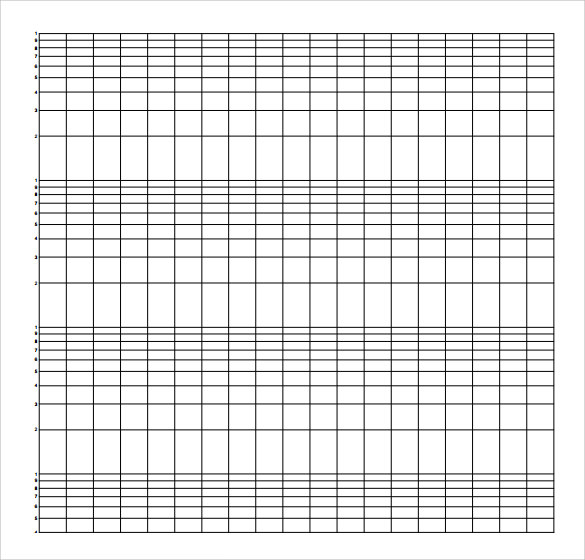# Semi-Log Graph Paper Template Printable PDF

You can look for the semi-log graph paper templates here available for free download in a printable format. Semi Log graph paper is one of the types of graph papers that are used by students of science and engineering.

In a semi-log graph, the y-axis is logarithmic, which means the separation between the ticks on the graph is proportional to the logarithm of numbers. The x-axis has a linear scale, which means the ticks are evenly spaced. Semi-log quadrille is used for decoding logarithmic equations in maths and science. It is mainly used by engineering students. This is used where the data on one axis is less than the data on the other axis.

A semi-log graph is useful when graphing exponential functions. Consider a function of the form y = bax. When graphed on semi-log paper, this function will produce a straight line with slope log (a) and y-intercept b.

Look for the free printable graph paper templates here and download a suitable one for your projects or assignments. You can use these templates for your use. You can take a print-out of this graph paper after downloading it from here. As you can see, all the templates are available in high-quality only will give you a nice print-out for your personal and professional use.

## Semi-Log Graph Paper Printable

It is important to note that Semi-log quadrille paper has one linear axis and another log axis, it is used for data where the progress of one variable is very large and is not linear than the other. This type of graph paper comes in handy when it comes to exponential functions.